Multiple Choice
Identify the choice that best completes the statement or answers the question.

1.

Geometry – Congruency and Similarity – RIT 211 – 220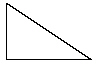Which figure is similar to the given figure?
 a.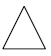d.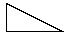b.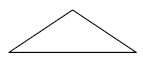e.c.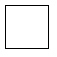2.

Triangle ABC is congruent to triangle RST.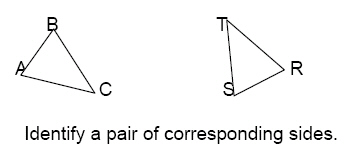a. AB and TR d. TR and TS b. BC and ST e. AB and TS c. AB and BC

3.

Geometry – Properties and Terminology – RIT 211 – 220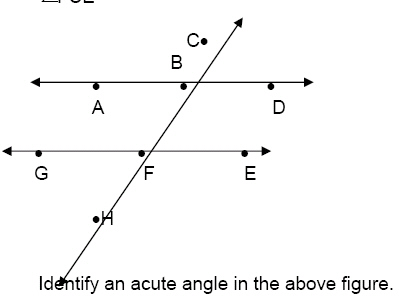a.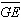d.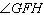b.e.AB c.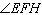4.a. A and B d. D and B b. A and C e. A and D c. B and C

5.

Triangle ABC is similar to Triangle LMN.a.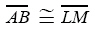d.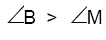b.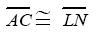e.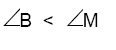c.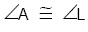6.

Geometry – Shapes and Figures – RIT 211 – 220Which of the following represents a face on the cube?
 a. point A d. point F b. plane ABCD e. segment DE c. segment AD

7.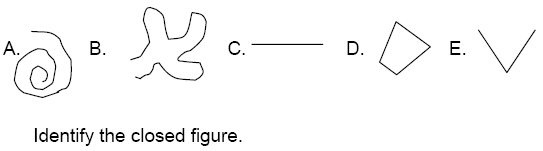a. A d. D b. B e. E c. C

8.

What is the name of a polygon with six sides?
 a. triangle d. hexagon b. quadrilateral e. octagon c. pentagon

9.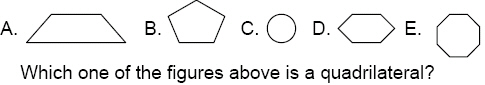a. A d. D b. B e. E c. C

10.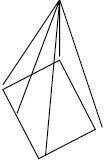How many vertices does a rectangular pyramid have?
 a. 4 d. 7 b. 5 e. 8 c. 6

11.

Geometry – Symmetry and Transformations – RIT 211 – 220
Identify the type of transformation.a. translation d. symmetry b. rotation e. congruent c. reflection

12.

Geometry – Congruency and Similarity – RIT 221 – 230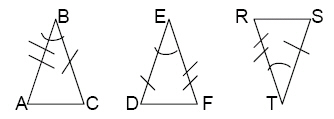Which congruency statement is written correctly?
 a. .ABC . EDF d. .ABC . .DEF b. .ABC . RST e. .STR . .DFE c. .DEF . .STR

13.

Geometry – Properties & Terminology – RIT 221 – 230Which of the following is a diameter of the circle?
 a.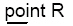d.b.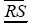e.c.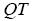14.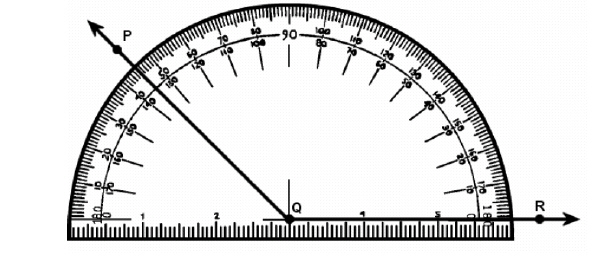a. 45 d. 115 b. 135 e. 90 c. 180

15.

Which pair of angles is supplementary?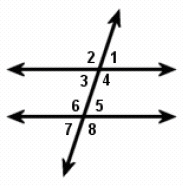a.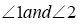d.b.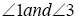e.c.16.

Which pair of angles are vertical angles?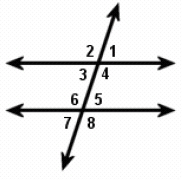a.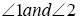d.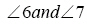b.e.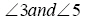c.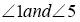17.

Geometry – Shapes and Figures – RIT 221 – 230

Which of the following has a rectangular base and four triangular faces?
 a. cube d. rectangular pyramid b. rectangular prism e. triangular pyramid c. triangular prism

18.

Which type of polygon has exactly one pair of opposite sides parallel?
 a. triangle d. parallelogram b. square e. rhombus c. trapezoid

19.

Measurement – Angle Measure – RIT 211 – 220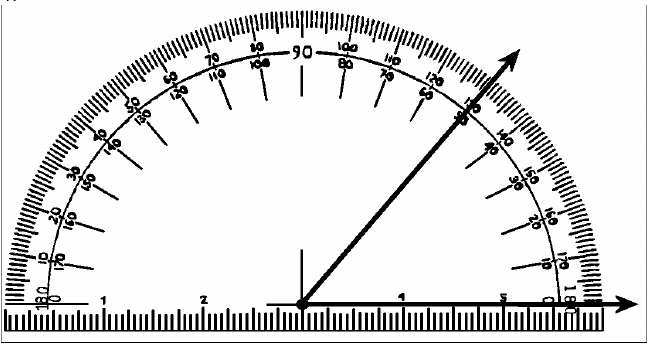What is the measure of the angle shown?
 a.d.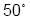b.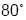e.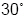c.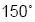20.

Measurement – Area, Perimeter, Circumference – RIT 211 – 220
Calculate the area of this shape.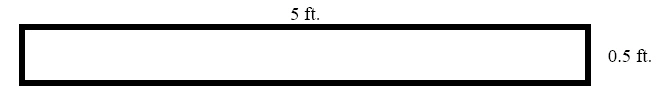a.d.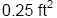b.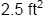e.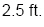c.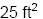21.

What happens to the perimeter when the length of a rectangle is
shortened?
 a. The perimeter stays the same. d. The perimeter is decreased by half. b. The perimeter becomes greater. e. The perimeter is increased by half. c. The perimeter becomes smaller.